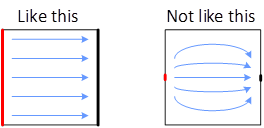# SHEET RESISTANC

Units = Ohms per Square (Ω/sq) or (Ω/□)

## Basic Concept

The resistance of one square of material, with a uniform thickness, is the same, regardless of size.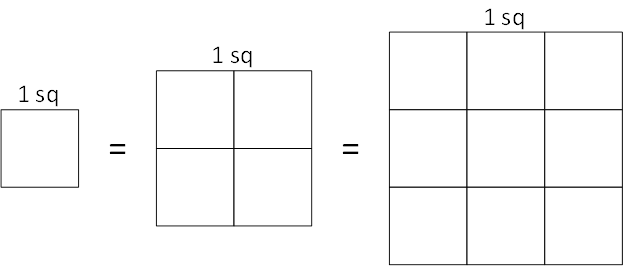## The Math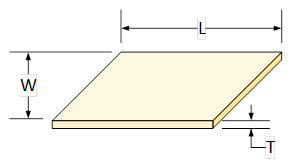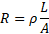Where:

R = Resistance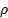= Material Resistivity

W = Width

L = Length

T = Thickness

A = Cross sectional Area = W × TNotice that if length and width are equal, then that term goes to 1 and only the resistivity and thickness matter.

## Measurements

Sheet resistance measurements must be made with connections across the entire side of the square